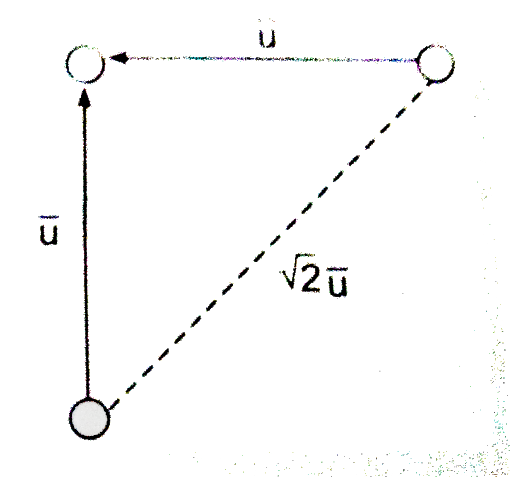Collision cross-section is an area of an imaginary sphere of radiu | FiloClass 11

Chemistry

Physical Chemistry

States of Matter551

Collision cross-section is an area of an imaginary sphere of radius around the molecule within which the centre of another molecule cannot penetrate.
The volume swept by a single molecule in unit time iswhere is the average speed
If is the number of molecules per unit volume, then the number of molecules within the volume V is

Hence, the number of collision made by a single molecule in unit time will be

In order to account for the movements of all molecules, we must consider the average velocity along the line of centres of two coliding molecules instead of the average velocity of a single molecule . If it is assumed that, on an average, molecules collide while approaching each other perpendicularly, then the average velocity along their centres is as shown below.Number of collision made by a single molecule with other molecule per unit time is given by

Z_(1)Z_(1)=(1)/(2)(Z_(1)N^(**))or Z_(11)=(1)/(2)(sqrt(2)pisigma^(2)overline(u)N^(**))N^(**)=(1)/(sqrt(2))pisigma^(2)overline(u)N^(**2)Z_(12)Z_(12)= pisigma _(12)^(2)(sqrt((8kT)/(pimu)))N_(1)N_(2)N_(1)N_(2)sigma_(12)mulambda=("Average distance travelled per unit time")/("NO. of collisions made by a single molecule per unit time")=(overline(u))/(Z_(1))or" "lambda=(overline(u))/(sqrt(2)pisigma^(2)overline(u)N^(**))implies(1)/(sqrt(2)pisigma^(2)overline(u)N^(**))
Calculate number of collision by one molecule per sec .

Solution: Solution:551Connecting you to a tutor in 60 seconds.